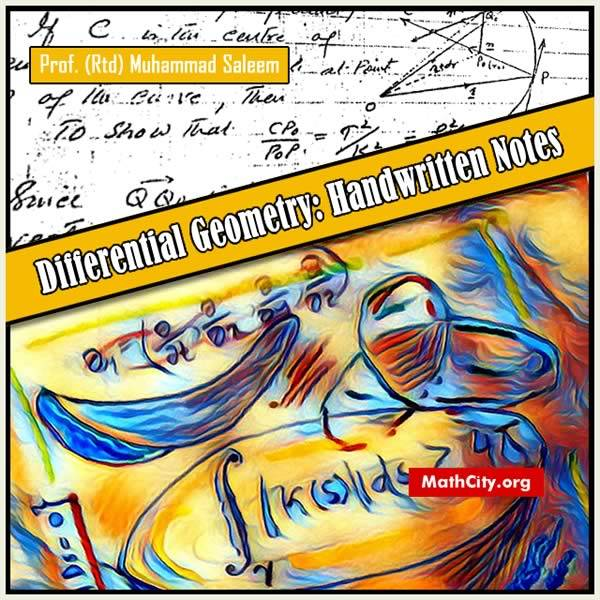# Differential Geometry: Handwritten NotesDifferential geometry is a discipline of mathematics that investigates the geometry of smooth objects and spaces, sometimes known as smooth manifolds. It investigates the geometric properties of curves and surfaces using the methods of differential and integral calculus, linear algebra, and multilinear algebra. Mathematical analysis and differential geometry are related concepts. These are the lecture notes of Prof. (Rtd.) Muhammad Saleem of University of Sargodha, Sargodha.

 Name Differential Geometry Handwritten Notes Prof. (Rtd) Muhammad Saleem 72 pages PDF 3.16 MB

Curves with torsion:
Curve, space curve, equation of tangent, normal plane, principal normal curvature, derivation of curvature, plane of the curvature or osculating plane, principal normal or binormal, rectifying plane, equation of binormal, torsion, Serret Frenet formulae, radius of torsion, the circular helix, skew curvature, centre of circle of curvature, spherical curvature, locus of centre of spherical curvature, helices, spherical indicatrix, evolute, involute.

Differential geometry of surfaces:
Surface, tangent plane and normal, equation of tangent plane, equaiton of normal, one parameter family of surfaces, characteristic of surface, envelopes, edge of regression, equation of edge of regression, developable surfaces, osculating developable, polar developable, rectifying developable.

• notes/differential_geometry_notes